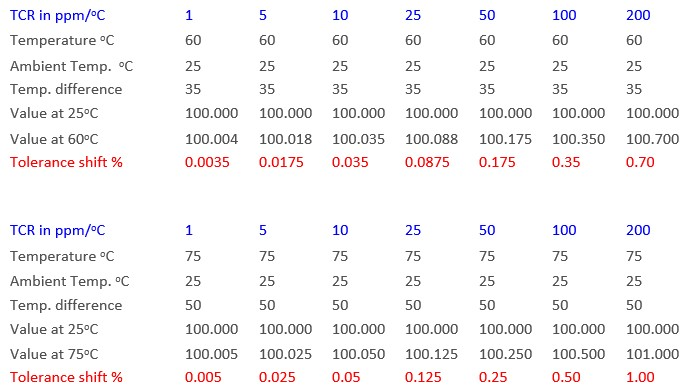Select Page

#### Minimize temperature coefficient of resistance (TCR)Temperature Coefficient of Resistance, or TCR, refers to how the value of a resistor will vary with changes in temperature.  This temperature change can be from changes in the environment around the resistor as well the resistor heating itself as it dissipates power.

Assuming a perfect tolerance resistor of 100 ohms at 25oC, the table below shows how the actual resistance value will change given different possible TCR choices.

RT = ((TCR * Ra * Td)/106) + Ra

Where:
RT = Resistance value at a given temperature
TCR = Temperature Coefficient of Resistance
Ra = Resistance value at ambient
Td = Change in TemperatureWheatstone Bridge and Scale Applications

As you can see, with a typical TCR of 50ppm and a perfect tolerance, the resistance value will shift 0.25% at a temperature rise of only 50oC.   This shift could be on either side depending on whether the TCR is positive or negative.   Another way to think about the situation is that if the design criteria calls for a resistance tolerance of 0.1% then to remain within the design limits you should specify a 10ppm or better TCR.

An example of an application where this may be important is the measurement of mass.  This is typically accomplished using a scale.  Scales may be used to measure the mass of gems, pharmaceuticals, or even larger items like construction equipment or bricks.  Whether the scale is in a laboratory or located on a shop floor it is important that the instrument be accurate and consistent regardless of the environmental temperature.  Most scales operate using the principal of a Wheatstone bridge as indicated in the image below.

Where:  Vo = (R3/(R3+R4) – R2/(R1+R2)) x Vi

The idea here is that when the ratio of R2/R1 equals the ratio of R3/R4 the voltage Vo will be zero.  In simple terms the scale works by making one of the resistors variable due to mass.   Anything other than a zero output means the bridge is out of balance.  The magnitude of this imbalance can then be correlated to mass.  Because the system relies on fixed ratios it is extremely important that the resistance values remain as stable as possible, since any variation could potentially make the bridge unbalanced.

Based on our knowledge of how TCR effects resistance value it is clear that a low TCR solution would be advantageous in these applications.  Riedon offers a number of solutions to meet the most stringent requirements.  Several of our low TCR solutions are presented below.